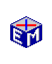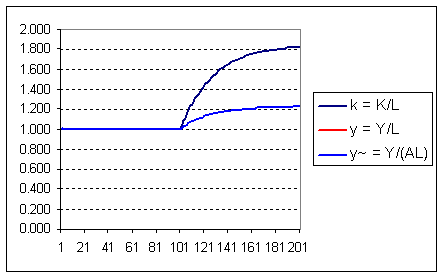The Solow Growth Model

The Solow Growth Model is described in detail at a level suitable for undergraduates in Charles I. Jones, Economic Growth, Second Edition, W.W. Norton and Company, 2002.

Production is given by the production function Y = Ka(AL)1-a, where Y is output, K is capital, L is labor, and A is a labor-augmenting technology factor.  The capital stock increases in a given period by the amount sY - dK, where s is the savings rate and d is the depreciation rate.  The labor force grows at rate n, and the technology factor A grows at rate g.

The Model Link below is an Excel spreadsheet that you can download (right click and select "save target as").  The spreadsheet implements a difference equation version of the differential equation form of the Solow Growth Model.The Solow Growth Model
"Save Target As" will make a copy.)
Exercises (printable pdf)Classic
Economic Models

Macroeconomics

Introduction
Overview of Macro Models

Microeconomics

Introduction
Overview of Micro Models

Resources